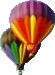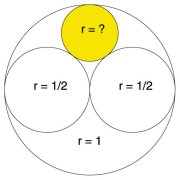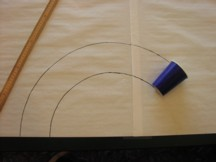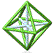Park City Mathematics Institute Geometrical Concepts from Constructions, Models, and Investigations Project Abstract Drafts of Project Files (password required)
Circle Packing
Celeste Williams
Circle Packing
Through a lunch conversation at PCMI, I found out about packing circles. The research mathematician I was talking to had found out about packing circles from a colleague of his that was intrigued by a high school geometry problem, which is below.

Packing circles have curvatures are always integers and when the curvature and radius are multiplied they produce a Gaussian integer. I have worked up a few classroom exercises that deal with finding the radius and curvature of the circles, as well as constructing tangent circles. There is an interesting, easy to read article about this to be found at http://www.sciencenews.org/200010421/bob18.aspFind the radius of a fourth circle nestled between the outer circle (radius = 1) and the two inner circles (each radius = 1/2).
Factoring Gaussian Integers
Part 1: A Geometric Model for Factoring Gaussian Integers
Part 2: Factoring Patterns in the Gaussian Plane
Steve Phelps
This article will be the result of my work as a member of the Geometry Working Group at the IAS/Park City Mathematics Institute during the summer of 2002. Part 1 details a method for multiplying and factoring Gaussian Integers by using the lattice points of circles. The method is applied to Gaussian Integers and ordinary integers. Part 2 describes the patterns of the factors of ordinary integers in the Gaussian plane. If an integer can be factored as the product of two Guassian Integers, then the factors will be located at the intersection points of two circles in a coaxal system.
The Puzzling Rhombic Dodecahedron
Joyce Frost & Peg Cagle
In On the Heavens, written in 350 B.C., Aristotle proclaimed that the regular tetrahedron fills space. He was mistaken. However, a particular tetrahedron, with dimensions derived from the cube, does fill space. This non-regular tetrahedron, and its closely related polyhedra, the cube, the rhombic dodecahedron, and the first stellation of the rhombic doecahedron, share dimensions and space filling properties. The rhombic dodecahedron derives its name from its twelve (dodeca) faces, all of which are congruent rhombi, each formed by joining two tetrahedra. In this article we will explore the connections between these related polyhedra and how to use their common dimensions to create a net or pattern to build three intriguing puzzles, which exploit their space filling properties.
Paper Cup Mathematics
Marilyn McArthur, Jerry Neidenbach, Susan Harvey
Imagine a discarded paper cup rolling on its side in a circular path. This observation provided the inspiration for the paper cup activity. Is the cup really rolling in a circle?

Students investigate this question and begin the activity by experimenting with paper cups. They devise methods to draw the path of the cup, which may include a compass and straightedge construction. The activity concludes with a problem that requires finding the area of a carousel platform and includes an investigation using Geometer's Sketchpad.Polygons and their Centers of Mass
Troy Jones     Abstract
Centers of Mass
Student page

• The center of mass as a balancing point
• Approach from a perspective that empowers students to construct the center of mass geometrically

• Balancing a segment (thin rod) of uniform density (midpoint)
• Balancing two points of equal mass (midpoint)
• Balancing two points of different masses (law of the lever)

Three models for polygons; area, vertex, and perimeter
Area model
• Balancing lines
• All balancing lines concur
• Special balancing lines facilitating geometric construction
• Why works? (thin strips)
• Extend to quadrilaterals, pentagons, etc. (diagonals)

Vertex model
• Difficulty with modeling
• Extend to other polygons by consecutively adding vertices

Perimeter model
• Balancing lines
• All balancing lines concur
• Special balancing lines
• Mathematics (angle bisector theorem, perimeter bisected)
• Extend to other polygons

Back to Geometry IndexPCMI@MathForum Home || IAS/PCMI Home© 2001 - 2018 Park City Mathematics Institute IAS/Park City Mathematics Institute is an outreach program of the School of Mathematics at the Institute for Advanced Study, Einstein Drive, Princeton, NJ 08540 Send questions or comments to: Suzanne Alejandre and Jim King